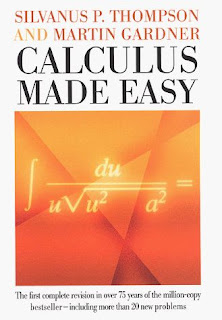Monday, November 16, 2009

Calculus Made Easy and Organic GrowthIn the latest Richard Dawkins’ book The Greatest Show on Earth: the Evidence for Evolution there is a mention (in a footnote in Chapter 10) of the book Calculus Made Easy by Silvanus P. Thompson and Martin Gardner. The reference came about because Dawkins was talking about how different characteristics of animals might change over one million years, like leg length. He imagines that a characteristic might increase by a factor of e over a million years, where e is the number 2.718281828 or the exponential function to the first power. In Dawkins’s argument the factor e was chosen for mathematical convenience but when reading Chapter XIV in Thompson’s book you can see why Dawkins chose it. From Thompson’s book: “the process of growing proportionately, at every instant, to the magnitude of that instant, some people call an exponential rate of growing. […] It might also be called the organic rate of growing: because it is characteristic of organic growth (in certain circumstances) that the increment of the organism in a given time is proportional to the magnitude of the organism itself.”

In Calculus Made Easy, Chapter 14 is titled On True Compound Interest and the Law of Organic Growth. In this chapter – quoted above- the idea of where e comes from is motivated by considering simple compound interest problems. In a nutshell, the general compound interest formula can be written as FV = PV (1 + 1/n)^n where a future value (FV) of a present value (PV) is related to the number of compounding operations (n). As n goes to infinity, (1 + 1/n)^n goes to the value e. It was the first time I considered how the value of e has a physical interpretation.

Also, while reading the part of Calculus Made Easy about derivatives something clicked and made sense about a bad experience I had with calculus while I was an undergraduate. Back 100 years ago I was taking an advanced calculus course and all we talked about were deltas and epsilons and taking limits. I remember being very lost. That’s the rigorous approach to derivatives developed by Karl Weierstrass (1815-1897) in the mid-nineteenth century. Before that, the approach used was something called infinitesimals – which is the approach taken by Thompson for motivating the concept of a derivative. It makes more sense to me and I wish I knew this all those years ago. (I could have been a very different person by now.) Infinitesimals were resurrected in the 1960s and today are called non-standard analysis.

1 comment:

1.the only word i understood in this summary is "easy."

I'm a huge dawkins fan, so Ya, I'll get right on reading this book. It would definitely help me with insomnia!
;)

All comments go through a moderation process. Even though it may not look like the comment was accepted, it probably was. Check back if you asked a question. Thanks!